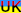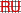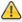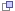УкраїнськаРоссийскийThis page is frozen,actual one here.

# The module <FLibMath> of the subsystem "Specials"

 Module: FLibMath Name: Math function's lib Type: Specials Source: spec_FLibMath.so Version: 0.6 Author: Roman Savochenko, Maxim Lysenko (2009-2010) Description: Provides the library of standard mathematical functions. License: GPL

Contents

## Introduction

Special FLibMath module provides the library of standard mathematical functions into the system.

To address the functions of the library you can use static call address "Special.FLibMath.{Func}()" or dynamic "SYS.Special.FLibMath["{Func}"].call()", "SYS.Special.FLibMath.{Func}()". Where {Func} — function identifier in the library.

## 1. Functions

Table 1 provides a description of each function of the library. For each function the evaluation time of execution is measured. The measurement was made on a system with the following parameters: Athlon 64 3000 + (ALTLinux 3.0 (32bit)), by measuring the total time of execution of the function, while calling it 1000 times.

Table 1: The functions of the library of standard mathematical functions

 Id Name Description Time (micro-seconds) abs Module Math. function — the number module. 81 acos Anti-cosine Math. function — anti-cosine. 149 asin Anti-sine Math. function — anti-sine. 140 atan Anti-tangent Math. function — anti-tangent. 109 ceil Rounding up to a larger Math. function — rounding up to a larger integer. 96 cos Сosine Math. function — cosine. 93 cosh Hyperbolic cosine Math. function — hyperbolic cosine. 121 exp Exponent Math. function — exponent. 145 floor Rounding to the lower Math. function — rounding to the lower integer 95 if If Condition Condition function — "If". 92 lg Common logarithm Math. function — common logarithm. 168 ln Natural logarithm Math. function — natural logarithm. 185 pow Power Math. function — involution. 157 rand Random number Math. function — random number generator. 147 sin Sine Math. function — sine. 127 sinh Hyperbolic sine Math. function — hyperbolic sine. 199 sqrt The square root Math. function — the square root. 94 tan Tangent Math. function — tangent. 153 tanh Hyperbolic tangent Math. function — hyperbolic tangent. 177

## 2. User programming API

Some objects of the module provides functions for user's programming.

The object "Functions library" (SYS.Special.FLibMath)

• ElTp {funcID}(ElTp prm1, ...) — call the library function {funcID}. Return result of the called function.

The object "User function" (SYS.Special.FLibMath["funcID"])

• ElTp call(ElTp prm1, ...) — call the function with parameters <prm{N}>. Return result of the called function.